Next: Periodicity of the Fourier Up: Fourier analysis and resynthesis Previous: Fourier analysis and resynthesis   Contents   Index

Fourier analysis of periodic signals

Suppose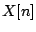is a complex-valued signal that repeats every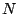samples. (We are continuing to use complex-valued signals rather than real-valued ones to simplify the mathematics.) Because of the period, the values offordeterminefor all integer values of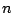.

Suppose further thatcan be written as a sum of complex sinusoids of frequency,,,,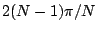. These are the partials, starting with the zeroth, for a signal of period. We stop at theth term because the next one would have frequency, equivalent to frequency, which is already on the list.

Given the values of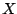, we wish to find the complex amplitudes of the partials. Suppose we want theth partial, where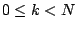. The frequency of this partial is. We can find its complex amplitude by modulatingdownwardradians per sample in frequency, so that theth partial is modulated to frequency zero. Then we pass the signal through a low-pass filter with such a low cutoff frequency that nothing but the zero-frequency partial remains. We can do this in effect by averaging over a huge number of samples; but since the signal repeats everysamples, this huge average is the same as the average of the firstsamples. In short, to measure a sinusoidal component of a periodic signal, modulate it down to DC and then average over one period.

Letbe the fundamental frequency for the period, and letbe the unit-magnitude complex number with argument: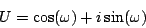Theth partial of the signalis of the form:where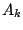is the complex amplitude of the partial, and the frequency of the partial is:We're assuming for the moment that the signalcan actually be written as a sum of thepartials, or in other words:By the heterodyne-filtering argument above, we expect to be able to measure eachby multiplying by the sinusoid of frequency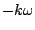and averaging over a period:This is such a useful formula that it gets its own notation. The Fourier transform of a signal, oversamples, is defined as:where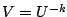. The Fourier transform is a function of the variable, equal totimes the amplitude of the input'sth partial. So farhas taken integer values but the formula makes sense for any value ofif we define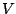more generally as:where, as before,is the (angular) fundamental frequency associated with the period.

SubsectionsNext: Periodicity of the Fourier Up: Fourier analysis and resynthesis Previous: Fourier analysis and resynthesis   Contents   Index
Miller Puckette 2006-12-30